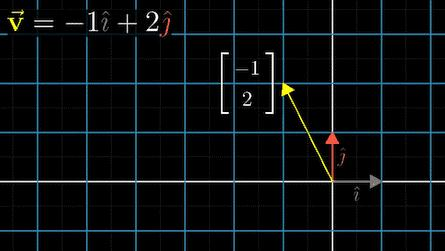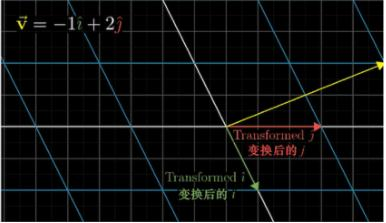矩阵乘法的几何理解

矩阵乘法

$\begin{bmatrix}1&3\\-2&0\end{bmatrix}\begin{bmatrix}-1\\2\end{bmatrix}=\begin{bmatrix}5\\2\end{bmatrix}$==》特征值和特征向量

$\begin{bmatrix}1&3\\-2&0\end{bmatrix}\begin{bmatrix}-1\\2\end{bmatrix}=\lambda\begin{bmatrix}-1\\2\end{bmatrix}$

$Mv=\lambda v$